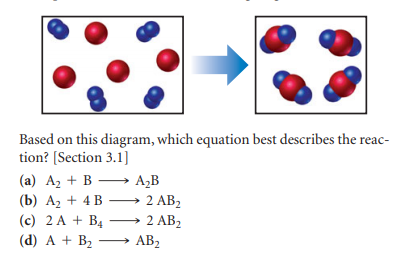×
Get Full Access to Chemistry: The Central Science - 12 Edition - Chapter 3 - Problem 1e
Get Full Access to Chemistry: The Central Science - 12 Edition - Chapter 3 - Problem 1e

×

# Visualizing ConceptsThe reaction between reactant A (blueISBN: 9780321696724 27

## Solution for problem 1E Chapter 3

Chemistry: The Central Science | 12th Edition

• Textbook Solutions
• 2901 Step-by-step solutions solved by professors and subject experts
• Get 24/7 help from StudySoup virtual teaching assistantsChemistry: The Central Science | 12th Edition

4 5 1 308 Reviews
23
0
Problem 1E

The reaction between reactant A (blue spheres) and reactant B (red spheres) is shown in the following diagram:Step-by-Step Solution:
Step 1 of 3

Chemistry 112 Lecture 1: Chapter 14 Chemical Kinetics In order for chemical reaction to occur: Molecules must COLLIDE with enough ENERGY to break and form bonds in perfect ORIENTATION that leads to atom rearrangement ­As chemical concentration increases, collisions increase ­As temperature rises, energy rises ­You cannot control or catalyze the molecules for perfect orientation Kinetics ­Study of rate of chemical reactions and how fast they proceed Reaction Rate ­ Helpful in determining reaction mechanism ­Equal to the change in concentration divided by change in time ­When determining change in anything, always subtract the initial value from the final value ­Useful because not all reactants are converted t

Step 2 of 3

Step 3 of 3

##### ISBN: 9780321696724

Chemistry: The Central Science was written by and is associated to the ISBN: 9780321696724. Since the solution to 1E from 3 chapter was answered, more than 427 students have viewed the full step-by-step answer. The answer to “?The reaction between reactant A (blue spheres) and reactant B (red spheres) is shown in the following diagram:” is broken down into a number of easy to follow steps, and 18 words. The full step-by-step solution to problem: 1E from chapter: 3 was answered by , our top Chemistry solution expert on 04/03/17, 07:58AM. This full solution covers the following key subjects: reactant, Spheres, reaction, diagram, based. This expansive textbook survival guide covers 49 chapters, and 5471 solutions. This textbook survival guide was created for the textbook: Chemistry: The Central Science, edition: 12.

Unlock Textbook Solution